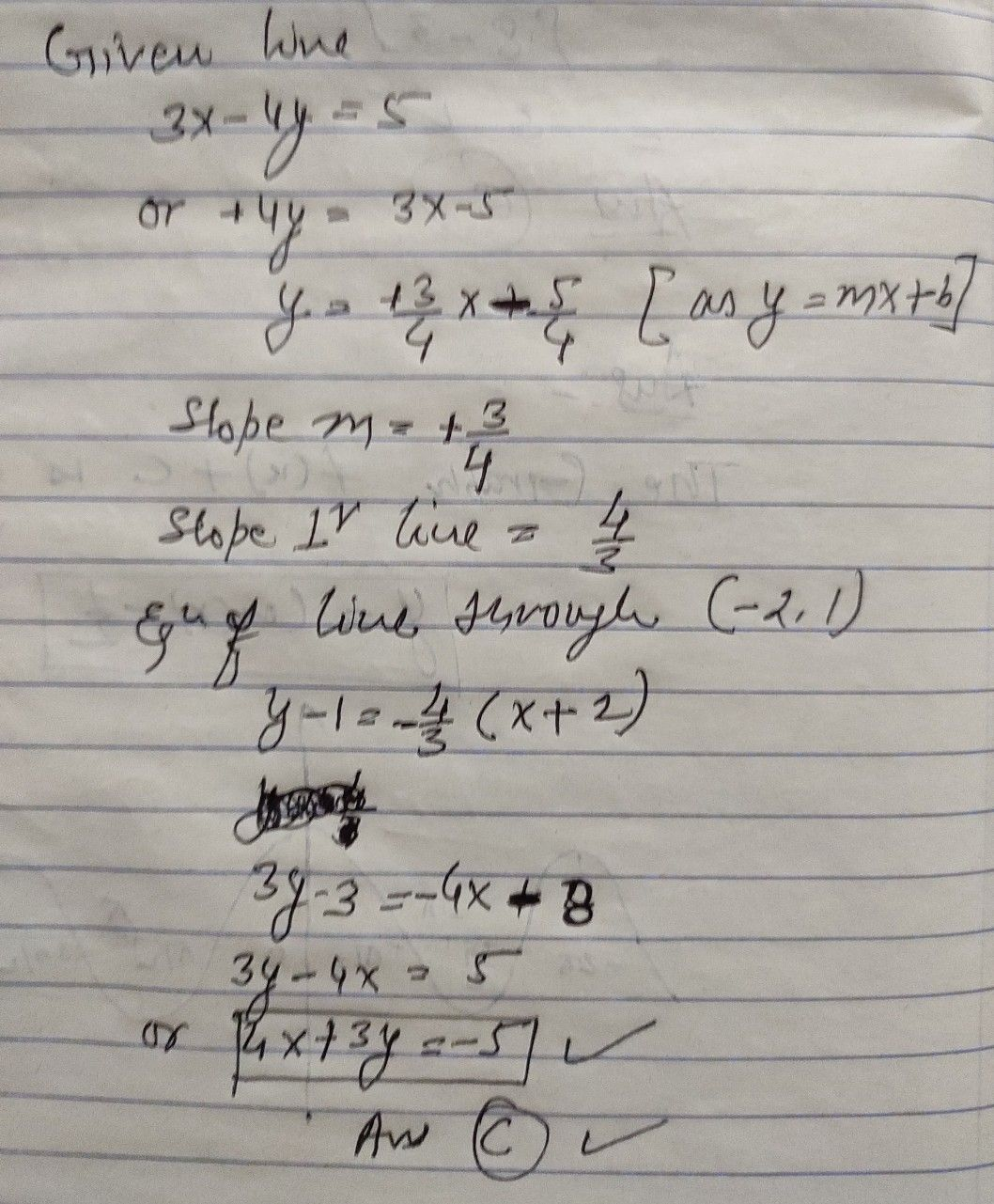Symbol
Problem$5°$ An equation of the straight line which is perpendicular to $3x-4y=5$ and passes through the point $\left(-2,1\right)$ is $A$ $4x+3y=-10$ $B$ $3x-4y=$ $10$ $C$ $4x+3y=$ $-5$ $D$ $-3x+4y=$ $10$ $E$ $4x-3y=$ $10$
English
SolutionQanda teacher - MksharmaI hope this helps youStudent